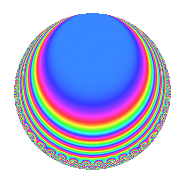# Properties

 Label 57.2.j.aLevel 57 Weight 2 Character orbit 57.j Analytic conductor 0.455 Analytic rank 0 Dimension 6 CM disc. -3 Inner twists 4

# Related objects

## Newspace parameters

 Level: $$N$$ = $$57 = 3 \cdot 19$$ Weight: $$k$$ = $$2$$ Character orbit: $$[\chi]$$ = 57.j (of order $$18$$ and degree $$6$$)

## Newform invariants

 Self dual: No Analytic conductor: $$0.455147291521$$ Analytic rank: $$0$$ Dimension: $$6$$ Coefficient field: $$\Q(\zeta_{18})$$ Coefficient ring: $$\Z[a_1, \ldots, a_{4}]$$ Coefficient ring index: $$1$$ Sato-Tate group: $\mathrm{U}(1)[D_{18}]$

## $q$-expansion

Coefficients of the $$q$$-expansion are expressed in terms of a primitive root of unity $$\zeta_{18}$$. We also show the integral $$q$$-expansion of the trace form.

 $$f(q)$$ $$=$$ $$q + ( -\zeta_{18}^{2} + 2 \zeta_{18}^{5} ) q^{3} + 2 \zeta_{18} q^{4} + ( -3 \zeta_{18} + 2 \zeta_{18}^{2} + 2 \zeta_{18}^{4} - 3 \zeta_{18}^{5} ) q^{7} -3 \zeta_{18}^{4} q^{9} +O(q^{10})$$ $$q + ( -\zeta_{18}^{2} + 2 \zeta_{18}^{5} ) q^{3} + 2 \zeta_{18} q^{4} + ( -3 \zeta_{18} + 2 \zeta_{18}^{2} + 2 \zeta_{18}^{4} - 3 \zeta_{18}^{5} ) q^{7} -3 \zeta_{18}^{4} q^{9} + ( -4 + 2 \zeta_{18}^{3} ) q^{12} + ( -1 - 3 \zeta_{18}^{2} - 3 \zeta_{18}^{3} - \zeta_{18}^{5} ) q^{13} + 4 \zeta_{18}^{2} q^{16} + ( -2 + 5 \zeta_{18}^{3} ) q^{19} + ( 4 - \zeta_{18} - 5 \zeta_{18}^{3} + 5 \zeta_{18}^{4} ) q^{21} + ( 5 \zeta_{18} - 5 \zeta_{18}^{4} ) q^{25} + ( 3 + 3 \zeta_{18}^{3} ) q^{27} + ( 6 - 6 \zeta_{18}^{2} - 2 \zeta_{18}^{3} + 4 \zeta_{18}^{5} ) q^{28} + ( -5 \zeta_{18} + \zeta_{18}^{2} - \zeta_{18}^{4} + 5 \zeta_{18}^{5} ) q^{31} -6 \zeta_{18}^{5} q^{36} + ( 4 \zeta_{18} - 4 \zeta_{18}^{2} + 3 \zeta_{18}^{4} + 7 \zeta_{18}^{5} ) q^{37} + ( 7 \zeta_{18} + 7 \zeta_{18}^{2} - 2 \zeta_{18}^{4} - 5 \zeta_{18}^{5} ) q^{39} + ( -6 - 6 \zeta_{18} - \zeta_{18}^{3} + 7 \zeta_{18}^{4} ) q^{43} + ( -8 \zeta_{18} + 4 \zeta_{18}^{4} ) q^{48} + ( -7 + 3 \zeta_{18} + 5 \zeta_{18}^{2} + 7 \zeta_{18}^{3} - 8 \zeta_{18}^{4} - 8 \zeta_{18}^{5} ) q^{49} + ( 2 - 2 \zeta_{18} - 8 \zeta_{18}^{3} - 6 \zeta_{18}^{4} ) q^{52} + ( -8 \zeta_{18}^{2} + \zeta_{18}^{5} ) q^{57} + ( -9 - 5 \zeta_{18}^{2} + 4 \zeta_{18}^{3} - 4 \zeta_{18}^{5} ) q^{61} + ( -3 + 6 \zeta_{18}^{2} - 6 \zeta_{18}^{3} + 3 \zeta_{18}^{5} ) q^{63} + 8 \zeta_{18}^{3} q^{64} + ( 9 + 9 \zeta_{18}^{2} - 7 \zeta_{18}^{3} - 2 \zeta_{18}^{5} ) q^{67} + ( 8 + \zeta_{18} + \zeta_{18}^{3} + 8 \zeta_{18}^{4} ) q^{73} + ( -5 + 10 \zeta_{18}^{3} ) q^{75} + ( -4 \zeta_{18} + 10 \zeta_{18}^{4} ) q^{76} + ( 7 + 10 \zeta_{18} - 10 \zeta_{18}^{3} - 7 \zeta_{18}^{4} ) q^{79} + ( -9 \zeta_{18}^{2} + 9 \zeta_{18}^{5} ) q^{81} + ( 8 \zeta_{18} - 2 \zeta_{18}^{2} - 10 \zeta_{18}^{4} + 10 \zeta_{18}^{5} ) q^{84} + ( 5 - \zeta_{18} - 11 \zeta_{18}^{2} + 6 \zeta_{18}^{3} + 2 \zeta_{18}^{4} + 6 \zeta_{18}^{5} ) q^{91} + ( 11 - 7 \zeta_{18} - 4 \zeta_{18}^{3} - 4 \zeta_{18}^{4} ) q^{93} + ( 6 \zeta_{18}^{2} - 3 \zeta_{18}^{5} ) q^{97} +O(q^{100})$$ $$\operatorname{Tr}(f)(q)$$ $$=$$ $$6q + O(q^{10})$$ $$6q - 18q^{12} - 15q^{13} + 3q^{19} + 9q^{21} + 27q^{27} + 30q^{28} - 39q^{43} - 21q^{49} - 12q^{52} - 42q^{61} - 36q^{63} + 24q^{64} + 33q^{67} + 51q^{73} + 12q^{79} + 48q^{91} + 54q^{93} + O(q^{100})$$

## Character Values

We give the values of $$\chi$$ on generators for $$\left(\mathbb{Z}/57\mathbb{Z}\right)^\times$$.

 $$n$$ $$20$$ $$40$$ $$\chi(n)$$ $$-1$$ $$\zeta_{18}$$

## Embeddings

For each embedding $$\iota_m$$ of the coefficient field, the values $$\iota_m(a_n)$$ are shown below.

For more information on an embedded modular form you can click on its label.

Label $$\iota_m(\nu)$$ $$a_{2}$$ $$a_{3}$$ $$a_{4}$$ $$a_{5}$$ $$a_{6}$$ $$a_{7}$$ $$a_{8}$$ $$a_{9}$$ $$a_{10}$$
2.1
 0.939693 + 0.342020i −0.766044 + 0.642788i 0.939693 − 0.342020i −0.173648 + 0.984808i −0.173648 − 0.984808i −0.766044 − 0.642788i
0 −1.11334 + 1.32683i 1.87939 + 0.684040i 0 0 −0.418748 0.725293i 0 −0.520945 2.95442i 0
14.1 0 1.70574 + 0.300767i −1.53209 + 1.28558i 0 0 −2.05303 3.55596i 0 2.81908 + 1.02606i 0
29.1 0 −1.11334 1.32683i 1.87939 0.684040i 0 0 −0.418748 + 0.725293i 0 −0.520945 + 2.95442i 0
32.1 0 −0.592396 + 1.62760i −0.347296 + 1.96962i 0 0 2.47178 4.28125i 0 −2.29813 1.92836i 0
41.1 0 −0.592396 1.62760i −0.347296 1.96962i 0 0 2.47178 + 4.28125i 0 −2.29813 + 1.92836i 0
53.1 0 1.70574 0.300767i −1.53209 1.28558i 0 0 −2.05303 + 3.55596i 0 2.81908 1.02606i 0
 $$n$$: e.g. 2-40 or 990-1000 Embeddings: e.g. 1-3 or 53.1 Significant digits: Format: Complex embeddings Normalized embeddings Satake parameters Satake angles

## Inner twists

Char. orbit Parity Mult. Self Twist Proved
1.a Even 1 trivial yes
3.b Odd 1 CM by $$\Q(\sqrt{-3})$$ yes
19.f Odd 1 yes
57.j Even 1 yes

## Hecke kernels

This newform can be constructed as the kernel of the linear operator $$T_{2}$$ acting on $$S_{2}^{\mathrm{new}}(57, [\chi])$$.